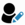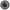Write a Program to print fibonacci series using recursion.# Search Interview QuestionsMore than 3000 questions in repository.There are more than 900 unanswered questions.Have a video suggestion.
Click Correct / Improve and please let us know.
Label / Company      Label / Company / TextSubmit Question

Java - Interview Questions and AnswersQ1. Write a Program to print fibonacci series using recursion.Core Java2017-08-24 14:41:45Ans. public class FibonacciUsingRecursion {
public static void main(String[] args) throws IOException{
getNextFibanocci(1,1);
}

static int getNextFibanocci(int a,int b){
if(a+b >= 100){
return 0;
} else {
System.out.println(a+b);
getNextFibanocci(b,a+b);
}

return 0;
}
}Help us improve. Please let us know the company, where you were asked this question :LikeDiscussCorrect / Improvefibonacci  recursionRelated QuestionsWhat will happen if we don't have termination statement in recursion ?Find Fibonacci first n numbers using recursion ?Write an Algorithm for Graph Traversal ? The Graph has a loop.Write a Program to print factorial of a number using recursionWrite a program to print sum of numbers between the start and end number

For example - Passing start number as 2 and end number as 10, it should print 2+3+4+5+6+7+8+9+10 = 54Write a program using Recursion to print multiplication of numbers between the start and end number

For example - Passing start number as 2 and end number as 10, it should print 2*3*4*5*6*7*8*9*10 = 3628800What is recursion in Java ?What will happen if we don't have termination statement in recursion ?Which of the two - iteration or recursion - is slower ?

## Help us and Others Improve. Please let us know the questions asked in any of your previous interview.

Any input from you will be highly appreciated and It will unlock the application for 10 more requests.